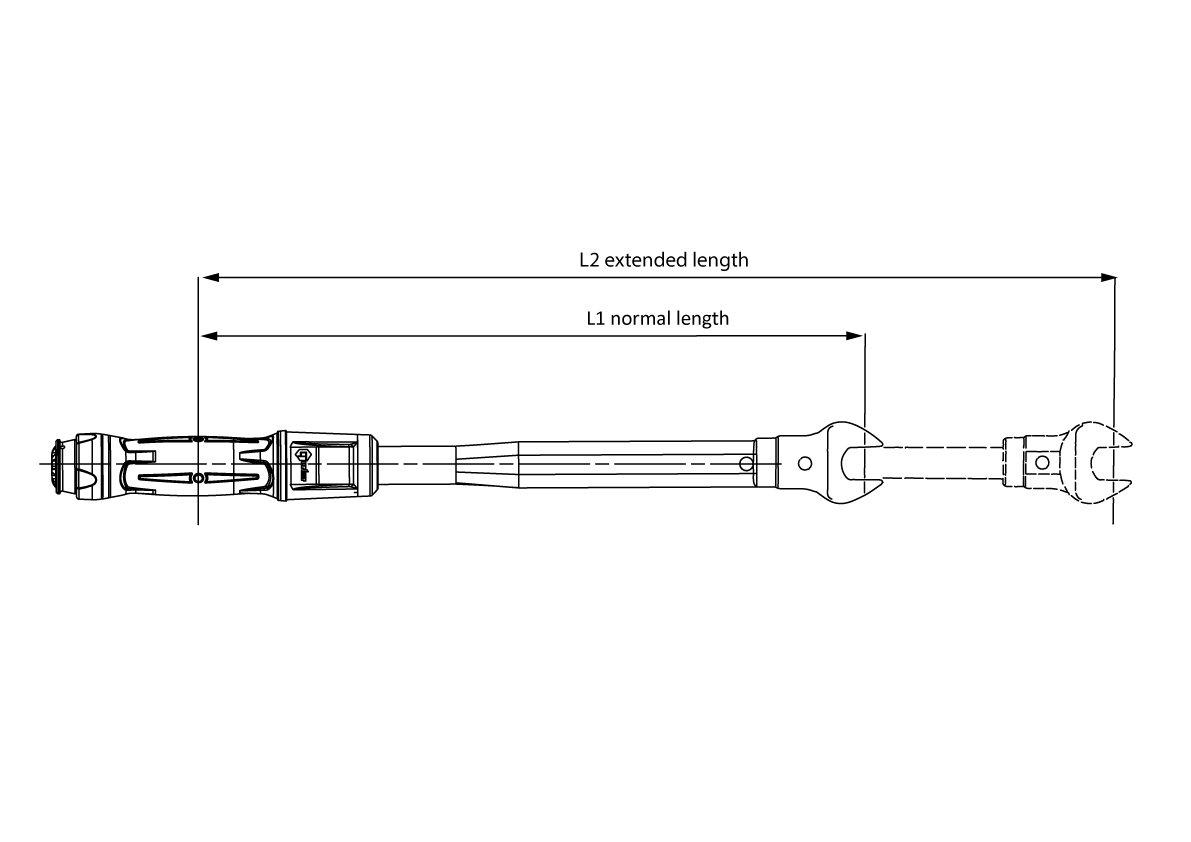Norbar Blog

02Jul

Introduction to Torque Part 5

02 Jul, 2019 | Return|

Torque Conversion Factors

The below formulas and information provide guidance on different conversion factors e.g. the effect of torque wrench extensions.

 Units to be converted S.I. Units Imperial Units Metric Units cN·m N·m ozf·in lbf·in lbf·ft Kgf·cm Kgf·m 1 cN·m = 1 0.01 1.416 0.088 0.007 0.102 0.001 1 N·m = 100 1 141.6 8.851 0.738 10.20 0.102 1 ozf·in = 0.706 0.007 1 0.0625 0.005 0.072 0.0007 1 lbf·in = 11.3 0.113 16 1 0.083 1.152 0.0115 1 lbf·ft = 135.6 1.356 192 12 1 13.83 0.138 1 kgf·cm = 9.807 0.098 13.89 0.868 0.072 1 0.001 1 kgf·m = 980.7 9.807 1389 86.8 7.233 100 1Formulae

Accepted formulae relating torque and tension, based on many tests are: -

For Imperial SizesThese formulae may be used for bolts outside the range of the tables.

Formula for Calculating the Effect of Torque Wrench Extensions

M1 = M2 x L1/L2

Where L1 is the normal length and L2 is the extended length, M1 is the set torque and M2 the actual torque applied to the nut.

Example

The required torque on the fastener is 130 N·m (M2) but what do you set on the torque wrench scale?

L1 = 500 L2 = 650

(units of length not important, this is ratio)

M1 = 130 x 500/650

M1 = 100